/  Technology   /  Accuracy, Recall, Precision, F1 Score in Python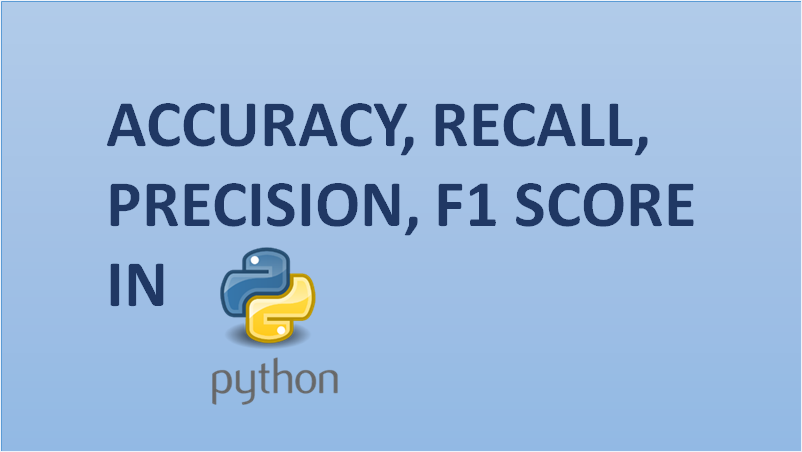## Accuracy, Recall, Precision, F1 Score in Python

Precision and recall are two crucial yet misjudged topics in machine learning. It often pops up on lists of common interview questions for data science positions. Describe the difference between precision and recall, explain what an F1 Score is, how important is accuracy to a classification model? It’s easy to get confused and mix these terms up with one another so I thought it’d be a good idea to break each one down and examine why they’re important.

For any machine learning model, we know that achieving a ‘good fit’ on the model is extremely crucial. This involves achieving the balance between underfitting and overfitting. However, when it comes to classification – there is another tradeoff that is precision-recall tradeoff.

## Accuracy

The formula for accuracy is pretty straight forward.But when dealing with classification problems we are attempting to predict a binary outcome. Is it fraud or not? Will this person default on their loan or not? Etc. So what we care about in addition to this overall ratio is number predictions that were falsely classified positive and falsely classified negative, especially given the context of what we are trying to predict.We have breakdown the accuracy formula even more.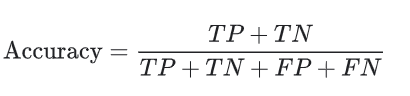Where TP = True Positive, TN = True Negatives, FP = False Positives and FN = False Negatives.

## Precision and Recall

Let me take in the confusion matrix and its parts here.

#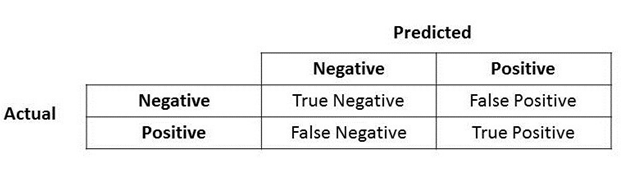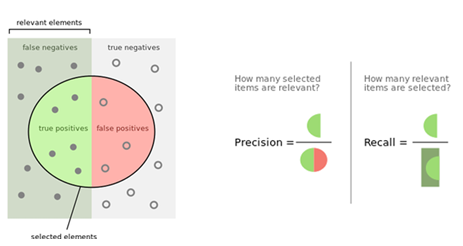Great! Now let us look at Precision first.What do you notice for the denominator? The denominator is actually the Total Predicted Positive! So, the formula becomes.True Positive + False Positive = Total Predicted Positive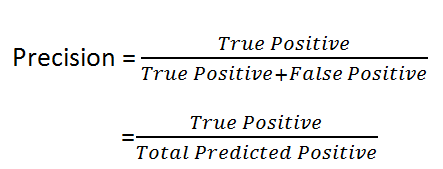Immediately, you can see that Precision talks about how precise/accurate your model is out of these predicted positive, what percent of them are actual positive.

Precision is a good measure to work out, when the costs of False Positive is high. For instance, in email spam detection a false positive means that an email that is non-spam (actual negative) has been identified as spam (predicted spam). The e-mail user might lose significant emails if the precision is not large for the spam detection model.

## Recall

So, let us put on the same logic for Recall. Recall how Recall is calculated.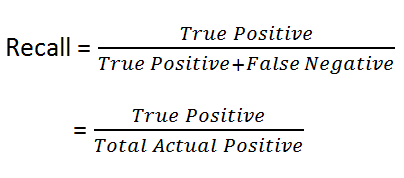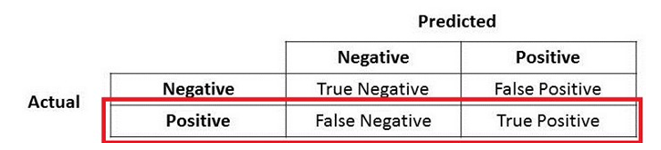True Positive + False Negative = Actual Positive

There you go! So, Recall actually calculates what percent of the Actual Positives our model capture through labeling it as Positive (True Positive). Applying an equivalent understanding, we know that Recall shall be the model metric we use to pick our best model when there is a high cost related to False Negative.

## F1 Score

You cannot evade the further measure, F1 which may be a function of Precision and Recall.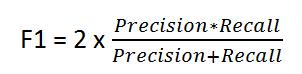F1 Score is required once you want to seek a balance between Precision and Recall.But…so what is the difference between F1 Score and Accuracy then? false positives and false negatives can be absolutely crucial to the study, while true negatives are often less import to whatever problem you’re trying to solve especially in a business setting. The F1 score tries to take this into account, giving more weight to false negatives and false positives while not letting large numbers of true negatives influence your score.

You realize that accuracy is not necessarily the end-all be-all of measurement for machine learning classification models. It’s really going to depend on what kind of problems you are trying to solve.